Courses

# Short Answer Type Questions (Part 1) - Triangles Class 10 Notes | EduRev

## Class 10 : Short Answer Type Questions (Part 1) - Triangles Class 10 Notes | EduRev

The document Short Answer Type Questions (Part 1) - Triangles Class 10 Notes | EduRev is a part of the Class 10 Course Class 10 Mathematics by VP Classes.
All you need of Class 10 at this link: Class 10

Q1.If one diagonal of a trapezium divides the other diagonal in the ratio of 1: 2. Prove that one of the parallel sides is double the other.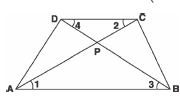Sol. Since ABCD is a trapezium,
∴ AB y cΔ ⇒ <1 = <2 and <3 = <4 (Alternate angle)
∴  Δ APB ~ Δ CPD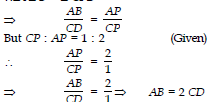Q2. In the figure, ABC is a D such that BD ⊥ AC and CE ⊥ AB. Prove that:
PD × BP = PC × EP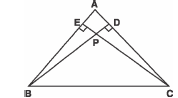Sol. In Δ BEP and Δ CPD, we have:
<BPE = <CPD
[Vertically opp. angles]
<BEP = <CDP [Each = 90°]
∴Using AA similarity, we have
Δ BEP ~ Δ CDP
∴Their corresponding sides are proportional,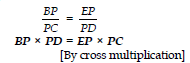⇒ BP × PD = EP × PC
[By cross multiplication]

Q3. AB is a line segment. PB ⊥ AB and QA ⊥ AB are such that PO = 5 cm and QO = 8 cm. If ar (D POB) = 250 cm2, then find the area of D QOA.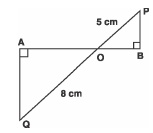Sol. In Δ QOA and Δ POB,
<QOA = <BOP
[Vertically opposite angles]
<QAO = <PBO [Each = 90°]
∴Using AA similarity, we have: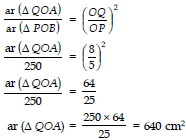Q4. In the figure, ABCD is a trapezium in which AB y CD. If D BOC ~ D AOD, then prove that AD = BC.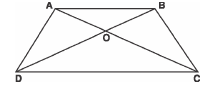Sol. We have a trapezium ABCD in which AB y DC.
Since Δ BOC   ~ Δ AOD [Given]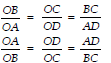.(1)
In Δ ODC and ΔOBA,
<COD = <AOB
[Vertically opp. angles]
<ODC = <OBA [Alt. angles]
∴ Using AA similarity, we have:
Δ ODC ~ Δ OBA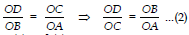...(2)
From (1) and (2)
=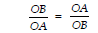⇒ OB × OB = OA × OA
⇒ OB2 = OA2  ⇒  OA = OB  ...(3)
From (1) to (3) we have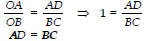Q5. P and Q are points on sides of AB and AC respectively of Δ ABC. If AP = 3 cm, PB= 9 cm, AQ = 5 cm and QC = 15 cm, then show that BC = 4 PQ.

Sol. We have Δ ABC in which P and Q are such that
AP = 3 cm,  PB = 9 cm
AQ = 5 cm,  QC = 15 cm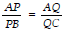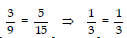i.e., PQ divides AB and AC in the same ratio
∴ PQ y BC
Now, in Δ APQ and Δ ABC
<P = <B [Corresponding angles]
<A = <A [Common]
⇒  Using AA similarity,
Δ APQ ~ Δ ABC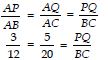⇒ =
[Œ AB = 3 + 9 = 12 cm and
AC = 5 + 15 = 20 cm]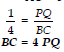Q6. On one of the longer sides PQ of a rectangle PQRS, a point O is taken such that
SO2 = PO· PQ
Prove that:
Δ POS ~ Δ OSR.
Sol.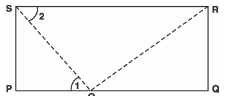We have a rectangle PQRS such that
SO2 = PO· PQ
i.e., SO × SO = PO × PQ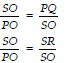⇒ =  ...(1)
[Œ PQ = SR, opp. sides of rectangle PQRS]
Now, in Δ POS and Δ OSR, we have: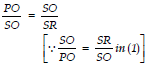<1 = <2 [OE PQ y SR,
opp. sides of a rectangle]
⇒ Using SAS similarity, we have
Δ POS ~ Δ OSR

Q7. Determine the length of the altitude AD of an isosceles D ABC in which AB = AC = 2a cm and BC = a cm.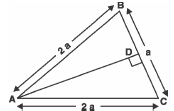Sol. We have Δ ABC in AD ⊥ BC and AB = AC = 2a. Also BC = a.
<B = <C [Opp. angles to equal sides of a D]
=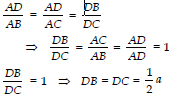⇒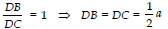Now in right Δ ABD, we have
⇒ AD2 = AB2 - BD2
= (AB + BD) (AB - BD)
=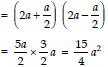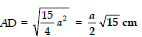Q8. In an equilateral triangle with side ‘a’, prove that its area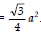.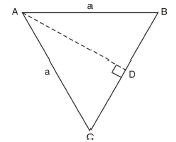Sol. We have Δ ABC such that
AB = BC = AC = a
Let us draw altitude AD ⊥ BC.
AC = AB [Each = a]
∴DC = DB =
⇒ AD2 = AB2 - DB2
= (AB + DB) (AB - DB)
=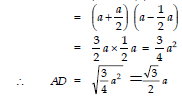Now, area of Δ  ABC =  1/2× Base × altitude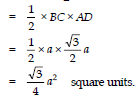Thus, the area of an equilateral triangle
=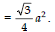Q9. In an equilateral triangle, prove that three times the square of one side is equal to four times the square of one of its altitudes.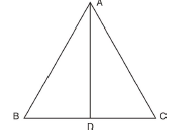Sol.We have Δ ABC in which AB = AC = CA and an altitude AD ⊥ BC.
AB = AB [Given]
Using RHS congruency, we have
⇒ DB = DC =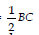...(1)
Now, in right Δ ADB, we have:
AB2 = AD2 + BD2 [Using Pythagoras Theorem]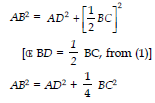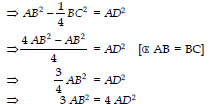⇒ 3 [Side of the equilateral triangle]
= 4 [Altitude]2.

Q10. ABC is a right triangle in which <C = 90° and CD ⊥ AB. If BC = a, CA = b, AB = c and CD = p, then prove that:
=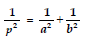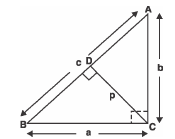Sol. We have a right Δ ABC such that <C = 90°.
Also, CD ⊥ AB
Now, ar (Δ ABC) =1/2 × Base × Height
=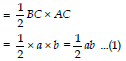...(1)
Also,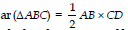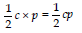. .. (2)
From (1) and (2), we have
=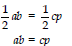Dividing throughout by abp, we have:
=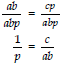Squaring both sides,
=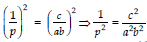=       ...(3)
Now, In right D ABC,
AB2 = AC2 + BC2
⇒ c2 = b2 + a2         ...(4)
∴ From (3) and (4), we get
=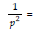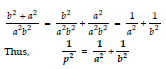Q11. ABC is a right triangle, right-angled at A, and D is the mid-point of AB. Prove that
BC2 = CD2 + 3 BD2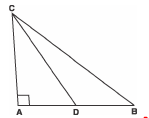Sol. We have a right Δ ABC in which <A = 90°
∴Using Pythagoras Theorem, we have:
BC2 = AB2 + AC2   ...(1)
Again, Δ ACD is right D, <A = 90°
...(2) [Using Pythagoras Theorem]
Subtracting (2) from (1), we get
BC2 - CD2 = AB2 - AD2 ...(3)
Since D is the mid-point of AB
∴2 BD = AB  and  AD = BD ...(4)
From (3) and (4), we have:
∴BC2 - CD2 = (2 BD)2 - (BD)2
= 4 BD2 - BD2
BC2 = CD2 + 3 BD2

Q12. In the figure, O is any point inside a rectangle ABCD such that OB = 6 cm, OD = 8 cm and OA = 5 cm. Find the length of OC.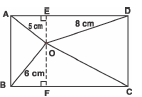Sol. Let us draw EOF y AB ⇒ OE ⊥ AD and OF ⊥ BC
In Δ OFB
Œ <F = 90°
∴Using Pythagoras theorem, we have:
CB2 = OF2 + BF2 ...(1)
In Δ OED
<E = 90°
∴Using Pythagoras theorem, we have:
OD2 = OE2 + DE2 ...(2)
Adding (1) and (2), we get
OB2 + OD2 = OF2 + BF2 + OE2 + DE2
= OF2 + AE2 + OE2 + CF2
[Œ BF = AE and CF = DE]
= (OF2 + CF2) + (OE2 + AE2)
= OC2 + OA2
= OC2 + 52
⇒ 62 + 82 = OC2 + 52
⇒ 36 + 64 = OC2 + 25
⇒ OC2 = 36 + 64 - 25 = 75
⇒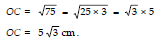Thus OC = 5√3cm.
Q13. In the figure, if AD ⊥ Bc,  then prove that:
AB
2 + CD2 = AC2 + BD2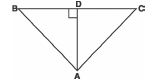∴ AD2 = AC2 - CD2 .....(1) (Using Pythagoras Theorem)
Similarly, in D AbD,
⇒ AD2 = AB2 - DB2.....(2)
From (1) and (2), we have
AB2 - DB2 = AC2 - CD2
⇒ AB2 + CD2 = AC2 + BD2

Q14. In the given figure, AD ⊥ BC and BD =  CD. Prove that: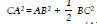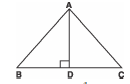Sol. BD = 1/2 CD
∴ 3 BD = CD
Since BD + DC = BC
∴ BD + 3 BD = BC
⇒ 4 BD = BC
⇒ BD = 1/4 BC
⇒ CD = 3/4 BC
Now, in right Δ ADC, < D = 90°
By Pythagoras theorem, we get
CA2 = AD2 + CD2  ...(1)
AD2 = AB2 - BD2  ...(2)
From (1) and (2),
CA2 = AB2 - BD2+ CD2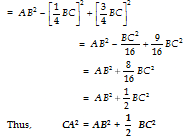Q15. In the given figure, M is the mid-point of side CD of parallelogram ABCD. The line BM is drawn intersecting AC at L, and AD produce D at E. Prove that EL = 2 BL.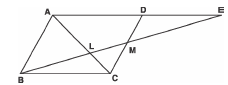Sol. We have parallelogram ABCD in which M is the mid point of CD.
In Δ EMD and Δ BMC
MD = MC [Œ M is mid-point of CD]
<EMD = <CMB
[Vertically opposite angles]
<MED = <MBC
[Alternate interior angles]
∴  Δ BMC ≌   Δ EMD     [AAS congruency]
⇒ BC = ED  ⇒  AD = ED    ...(1)
[Œ BC = AD,  opposite sides of parallelogram]
Now, in   Δ AEL and   Δ CBL
<AEL = <CBL [Alternate interior angles]
<ALE = <CLB
[Vertically opposite angles]
∴ By AA similarity, we have: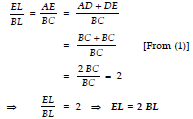Q16. In the given figure, Δ ABC is right-angled at C and DE ^ AB. Prove that Δ ABC ~ Δ ADE and hence find the length of AE and DE.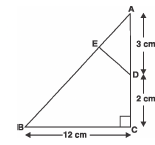Sol.In Δ ABC and Δ ADE, we have:<A = <A [Common]
<C = <E [Each = 90°]
∴ Δ ABC ~ Δ ADE [AA Similarity]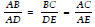...(1)In right Δ ABC, <C = 90°Using Pythagoras theorem, we have:
AB2 = BC2 + AC2
= 122 + 52
= 144 + 25 = 169
⇒AB = √169 = 13 cm
Now, from (1), we get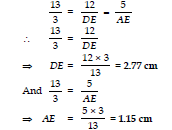⇒ DE = = 2.77 cmAnd =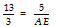⇒ AE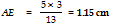Q17. In the given figure, DEFG is a square and <BAC = 90°. Show that DE2 = BD × EC.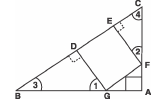Sol. In Δ DBG and Δ ECF
<3 + <1 = 90° = <3 + <4
∴<3 + <1 = <3 + <4
⇒ <1 = <4
<D = E = 90°
∴Using AA similarity, we have: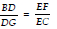BD × EC = EF × DGBut DG = EF = DE
∴BD × EC = DE × DE
⇒ BD × EC = DE2
Thus, DE2 = BD × EC

Q18. In the figure, AD ⊥ BC and BD =  CD. Prove that 2 CA2 = 2 AB2 + BC2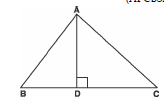Sol. Œ BD = 1/3 CD
⇒ 3 BD = CD
∴BC = BD + DC
⇒ BC = BD + 3 BD
⇒ BC = 4 BD ...(1)
And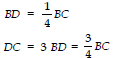From (2) ...(4)In right Δ ADC,
Using Pythagoras theorem,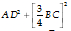From (3) ...(4)In right Δ ADB
Using Pythagoras theorem,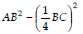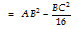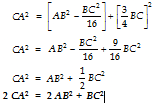Q19.If the diagonals of a quadrilateral divide each other proportionally, prove that it is a trapezium.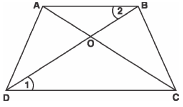Sol. We have a quadrilateral ABCD such that its diagonals intersect at O and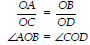<AOB = <COD [Vertically opposite angles]∴ Using SAS similarity, we have
Δ AOB ~ Δ COD
⇒ Their corresponding angles are equal.
i.e., <1 = <2
But they form a pair of int. alt. angles.
⇒ AB y DC
⇒ ABCD is a trapezium.

Q20. Two triangles ABC and DBC are on the same base BC and on the same side of BC in which
<A = <D = 90°. If CA and BD meet each other at E, show that
AE· EC = BE· ED
Sol.
We have right Δ ABC and right Δ DBC on the same base BC such that
<A = <D = 90°
In Δ ABE and Δ DCE
<A = <D = 90°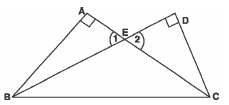<1 = <2 [Vertically opp. angles]
∴ Using AA similarity, we have:
Δ ABE ~ Δ DCE
⇒ Their corresponding sides are proportional.
⇒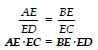⇒ AE· EC = BE· ED
Q21. E is a point on the side AD produced of a parallelogram ABCD and BE intersects CD at F. Show that
Δ ABE ~ Δ CFB
Sol.
We have parallelogram ABCD
In Δ ABE and Δ CFB, we have
<A = <C
[Opposite angles of parallelogram]
<AEB = <EBC
∴ Using AA similarity, we get
Δ ABE ~ Δ CFB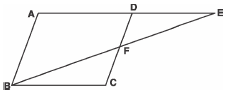Q22. In Δ ABC, if AD is the median, then show that AB2 + AC2 = 2 [AD2 + BD2].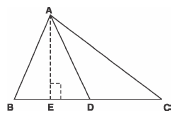Sol. AD is a median,
∴  BD = DC
Let us draw AE ≌ BC
Now, in rt. Δs AEB and AEC, we have
AB2 = BE2 + AE2 ...(1)
AC2 = CE2 + AE2 ...(2)
AB2 + AC2 = BE2 + AE2 + CE2 + AE2
= (BD - ED)2 + AE2 +
(CD + DE)2 + AE2
= 2AE2 + 2ED2 + BD2 + CD2
= 2 [AE2 + ED2] + BD2 + BD2
[BD = CD]
Thus,  AB2 + AC2 = 2 [AD2 + BD2]

Q23. Triangle ABC is right angled at B and D is the mid point of BC. Prove that:
AC2 = 4 AD- 3 AB2
Sol.
D is the mid-point of BC.
∴ BC = 2 BD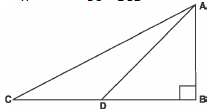Now, in Δ ABCAC2 = BC2 + AB2
= (2 BD)2 + AB2
= 4 BD2 + AB2 ...(1)
In right Δ ABD
Using Pythagoras theorem,
⇒ BD2 = AD2 - AB2 ...(2)
From (1) and (2), we get
AC2 = 4 [AD2 - AB2] + AB2
⇒ AC2 = - 4 AB2 + 4 AD2 + AB2
⇒ AC2 = - 3 AB2 + 4 AD2
or AC2 = 4 AD2 - 3 AB2

Offer running on EduRev: Apply code STAYHOME200 to get INR 200 off on our premium plan EduRev Infinity!

132 docs

,

,

,

,

,

,

,

,

,

,

,

,

,

,

,

,

,

,

,

,

,

;